# MA.912.AR.1.1Export Print
Identify and interpret parts of an equation or expression that represent a quantity in terms of a mathematical or real-world context, including viewing one or more of its parts as a single entity.

### Examples

Algebra 1 Example: Derrick is using the formula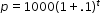to make a prediction about the camel population in Australia. He identifies the growth factor as (1+.1), or 1.1, and states that the camel population will grow at an annual rate of 10% per year.

Example: The expression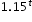can be rewritten as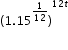which is approximately equivalent to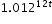. This latter expression reveals the approximate equivalent monthly interest rate of 1.2% if the annual rate is 15%.

### Clarifications

Clarification 1: Parts of an expression include factors, terms, constants, coefficients and variables.

Clarification 2: Within the Mathematics for Data and Financial Literacy course, problem types focus on money and business.

General Information
Subject Area: Mathematics (B.E.S.T.)
Strand: Algebraic Reasoning
Status: State Board Approved

## Benchmark Instructional Guide

• Coefficient
• Expression
• Equation

### Vertical Alignment

Previous Benchmarks

Next Benchmarks

### Purpose and Instructional Strategies

In grade 8, students generated and identified equivalent linear expressions, and solved multi-step problems involving linear expressions within real-world contexts. In Algebra I, students generate and interpret equivalent linear, absolute value, quadratic and exponential expressions and equations. In later courses, students will identify and interpret other functional (exponential, rational, logarithmic, trigonometric, etc.) expressions and equations.
• Instruction includes making the connection to linear, absolute value, quadratic and exponential functions.
• Students should be able to identify factors, terms, constants, coefficients and variables in expressions and equations.
• Go beyond these popular parts of an expression and equation: the growth/decay factor in exponential functions, rate of change in linear functions, interest, etc.
• Look for opportunities to interpret these components in context – make these discussions part of daily instruction.

### Common Misconceptions or Errors

• Students may not be able to identify parts of an expression and equation or interpret those parts within context. Ensure these are embedded throughout instruction and discussions.
• For example, building in questions to identify these parts and discussing their connection to the context in which they represent in a routine way will help students to make these connections.

### Strategies to Support Tiered Instruction

• Teacher facilitates discussions which include questions and clarifications to identify the connections of expressions and equations to the context of problems.
• Instruction provides opportunities to increase understanding of vocabulary terms.
• For example, instruction may include a vocabulary review using a chart shown.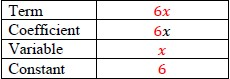• Teacher provides students with an expression or equation and allows them to match the parts to key vocabulary.
• For example, teacher can provide the word bank to identify the different parts of the equation shown.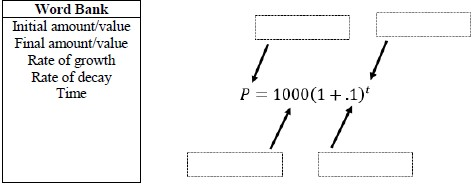• The algebraic expression ($n$ − 1)2 + (2$n$ − 1) can be used to calculate the number of symbols in each diagram below. Explain what $n$ likely represents, how the parts of this expression relate to the diagrams, and why the expression results in the number of symbols in each diagram.• Last weekend, Cindy purchased two tops, a pair of pants, and a skirt at her favorite store. The equation $T$ = 1.075$x$ can be used to calculate her total cost where $x$ represents the pretax subtotal cost of her purchase.
• Part A. In the equation $T$ = 1.075$x$, what does the number 1 represent? Explain using the context of Cindy’s situation.
• Part B. In the equation $T$ = 1.075$x$, what does the number 0.075 represent? Explain using the context of Cindy’s situation.

### Instructional Items

Instructional Item 1
• Identify the factors in the expression 2(3$x$ − 1) + 2(2$x$ + 2).

*The strategies, tasks and items included in the B1G-M are examples and should not be considered comprehensive.

## Related Courses

This benchmark is part of these courses.
1200310: Algebra 1 (Specifically in versions: 2014 - 2015, 2015 - 2022, 2022 and beyond (current))
1200320: Algebra 1 Honors (Specifically in versions: 2014 - 2015, 2015 - 2022, 2022 and beyond (current))
1200330: Algebra 2 (Specifically in versions: 2014 - 2015, 2015 - 2022, 2022 and beyond (current))
1200340: Algebra 2 Honors (Specifically in versions: 2014 - 2015, 2015 - 2022, 2022 and beyond (current))
1200370: Algebra 1-A (Specifically in versions: 2014 - 2015, 2015 - 2022, 2022 and beyond (current))
1200380: Algebra 1-B (Specifically in versions: 2014 - 2015, 2015 - 2022, 2022 and beyond (current))
1200400: Foundational Skills in Mathematics 9-12 (Specifically in versions: 2014 - 2015, 2015 - 2022, 2022 and beyond (current))
7912080: Access Algebra 1A (Specifically in versions: 2014 - 2015, 2015 - 2018, 2018 - 2019, 2019 - 2022, 2022 and beyond (current))
7912090: Access Algebra 1B (Specifically in versions: 2014 - 2015, 2015 - 2018, 2018 - 2019, 2019 - 2022, 2022 and beyond (current))
1200315: Algebra 1 for Credit Recovery (Specifically in versions: 2014 - 2015, 2015 - 2022, 2022 and beyond (current))
1200375: Algebra 1-A for Credit Recovery (Specifically in versions: 2014 - 2015, 2015 - 2022, 2022 and beyond (current))
1200385: Algebra 1-B for Credit Recovery (Specifically in versions: 2014 - 2015, 2015 - 2022, 2022 and beyond (current))
7912075: Access Algebra 1 (Specifically in versions: 2014 - 2015, 2015 - 2018, 2018 - 2019, 2019 - 2022, 2022 and beyond (current))
7912095: Access Algebra 2 (Specifically in versions: 2016 - 2018, 2018 - 2019, 2019 - 2022, 2022 and beyond (current))
1210305: Mathematics for College Statistics (Specifically in versions: 2022 and beyond (current))
1200388: Mathematics for Data and Financial Literacy Honors (Specifically in versions: 2022 and beyond (current))
1200384: Mathematics for Data and Financial Literacy (Specifically in versions: 2022 and beyond (current))
7912120: Access Mathematics for Data and Financial Literacy (Specifically in versions: 2022 - 2023, 2023 and beyond (current))

## Related Access Points

Alternate version of this benchmark for students with significant cognitive disabilities.
MA.912.AR.1.AP.1: Identify a part(s) of an equation or expression and explain the meaning within the context of a problem.

## Related Resources

Vetted resources educators can use to teach the concepts and skills in this benchmark.

## Formative Assessments

Interpreting Basic Tax:

Students are asked to interpret the parts of an equation used to calculate the total purchase price including tax of a set of items.

Type: Formative Assessment

Dot Expressions:

Students are asked to explain how parts of an algebraic expression relate to the number and type of symbols in a sequence of diagrams.

Type: Formative Assessment

What Happens?:

Students are asked to determine how the volume of a cone will change when its dimensions are changed.

Type: Formative Assessment

## Lesson Plans

Free Fall Clock and Reaction Time!:

This will be a lesson designed to introduce students to the concept of 9.81 m/s2 as a sort of clock that can be used for solving all kinematics equations where a = g.

Type: Lesson Plan

How much is your time worth?:

This lesson is designed to help students solve real-world problems involving compound and continuously compounded interest. Students will also be required to translate word problems into function models, evaluate functions for inputs in their domains, and interpret outputs in context.

Type: Lesson Plan

Efficient Storage:

The topic of this MEA is work and power. Students will be assigned the task of hiring employees to complete a given task. In order to make a decision as to which candidates to hire, the students initially must calculate the required work. The power each potential employee is capable of, the days they are available to work, the percentage of work-shifts they have missed over the past 12 months, and the hourly pay rate each worker commands will be provided to assist in the decision process. Full- and/or part-time positions are available. Through data analysis, the students will need to evaluate which factors are most significant in the hiring process. For instance, some groups may prioritize speed of work, while others prioritize cost or availability/dependability.

Type: Lesson Plan

Turning Tires Model Eliciting Activity:

The Turning Tires MEA provides students with an engineering problem in which they must work as a team to design a procedure to select the best tire material for certain situations. The main focus of the MEA is applying surface area concepts and algebra through modeling.

Type: Lesson Plan

## Original Student Tutorial

Identifying Parts of Linear Expressions:

Learn to identify and interpret parts of linear expressions in terms of mathematical or real-world contexts in this original tutorial.

Type: Original Student Tutorial

## Perspectives Video: Professional/Enthusiast

Base 16 Notation in Computing:

Listen in as a computing enthusiast describes how hexadecimal notation is used to express big numbers in just a little space.

Type: Perspectives Video: Professional/Enthusiast

## Perspectives Video: Teaching Idea

Programming Mathematics: Algebra, and Variables to control Open-source Hardware:

If you are having trouble understanding variables, this video might help you see the light.

Type: Perspectives Video: Teaching Idea

## STEM Lessons - Model Eliciting Activity

Efficient Storage:

The topic of this MEA is work and power. Students will be assigned the task of hiring employees to complete a given task. In order to make a decision as to which candidates to hire, the students initially must calculate the required work. The power each potential employee is capable of, the days they are available to work, the percentage of work-shifts they have missed over the past 12 months, and the hourly pay rate each worker commands will be provided to assist in the decision process. Full- and/or part-time positions are available. Through data analysis, the students will need to evaluate which factors are most significant in the hiring process. For instance, some groups may prioritize speed of work, while others prioritize cost or availability/dependability.

Turning Tires Model Eliciting Activity:

The Turning Tires MEA provides students with an engineering problem in which they must work as a team to design a procedure to select the best tire material for certain situations. The main focus of the MEA is applying surface area concepts and algebra through modeling.

## MFAS Formative Assessments

Dot Expressions:

Students are asked to explain how parts of an algebraic expression relate to the number and type of symbols in a sequence of diagrams.

Interpreting Basic Tax:

Students are asked to interpret the parts of an equation used to calculate the total purchase price including tax of a set of items.

What Happens?:

Students are asked to determine how the volume of a cone will change when its dimensions are changed.

## Original Student Tutorials Mathematics - Grades 9-12

Identifying Parts of Linear Expressions:

Learn to identify and interpret parts of linear expressions in terms of mathematical or real-world contexts in this original tutorial.

## Student Resources

Vetted resources students can use to learn the concepts and skills in this benchmark.

## Original Student Tutorial

Identifying Parts of Linear Expressions:

Learn to identify and interpret parts of linear expressions in terms of mathematical or real-world contexts in this original tutorial.

Type: Original Student Tutorial

## Perspectives Video: Professional/Enthusiast

Base 16 Notation in Computing:

Listen in as a computing enthusiast describes how hexadecimal notation is used to express big numbers in just a little space.

Type: Perspectives Video: Professional/Enthusiast

## Parent Resources

Vetted resources caregivers can use to help students learn the concepts and skills in this benchmark.

## Perspectives Video: Professional/Enthusiast

Base 16 Notation in Computing:

Listen in as a computing enthusiast describes how hexadecimal notation is used to express big numbers in just a little space.